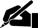# Thesis: Efficient Portfolio

Pages: 1 (349 words)  ·  Style: APA  ·  Bibliography Sources: 2  ·  File: .docx  ·  Level: College Senior  ·  Topic: Accounting - Personal Finance  ·  Buy for \$19.77

Efficient Portfolio

The weighted average expected return under the new scenario will be 14%. This is calculated as follows:

The standard deviation of returns under the new scenario is 24.5. We calculate the difference between each potential return and the mean. Thus,

(44-14) = 30; (14-14) = 0, and (14+16) = 30.

Each is squared, and then the squares are added together. So 302 = 900. This means that the sum of squares is 900 + 0 + 900 = 1800. This is divided by the number of data points, as follows:

This is the variance. To obtain the standard deviation we take the square root of 600 as follows:

The expected return is .333(12)+(.333)(4)+(.333)(-5.5) = 3.5

The standard deviation therefore is 12.38.

With half in T-bills the expected return is 3.75%. The standard deviation is therefore 6.015. The expected return improves because you reduce the downside risk with the T-bills. This also reduces the variability of the portfolio as well.

Problem

The expected return of this portfolio is as follows:

(.5)(15)+(.4)(10)+(.1)(6) = 12.1%… [END OF PREVIEW]

### Ordering Options:

1.  Buy Full Paper (1 Pages)

Perfectly formatted MS Word document!

or

2.  Write a NEW paper for you!Popular!

#### Markowicz the Objective of Modern Portfolio Theory Research Proposal…

Cite This Thesis:

APA Format

Efficient Portfolio.  (2009, September 25).  Retrieved November 21, 2019, from https://www.essaytown.com/subjects/paper/efficient-portfolio/71274

MLA Format

"Efficient Portfolio."  25 September 2009.  Web.  21 November 2019. <https://www.essaytown.com/subjects/paper/efficient-portfolio/71274>.

Chicago Format

"Efficient Portfolio."  Essaytown.com.  September 25, 2009.  Accessed November 21, 2019.
https://www.essaytown.com/subjects/paper/efficient-portfolio/71274.